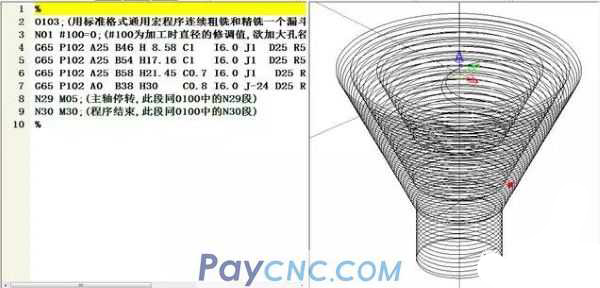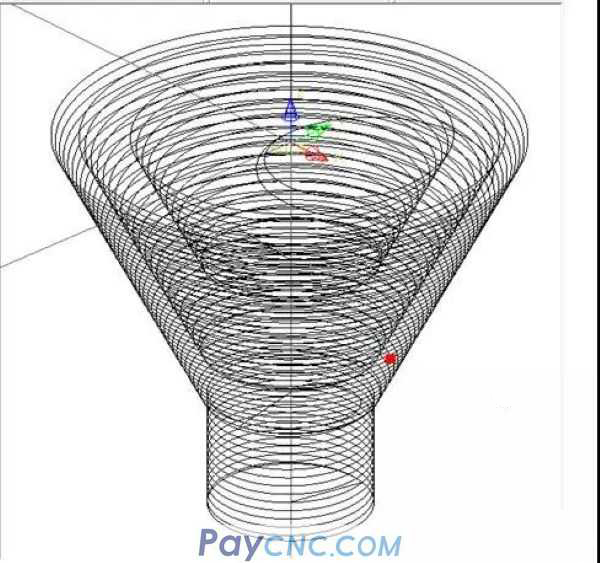The key point of logical reasoning in macro programming is: deriving the relationship between variables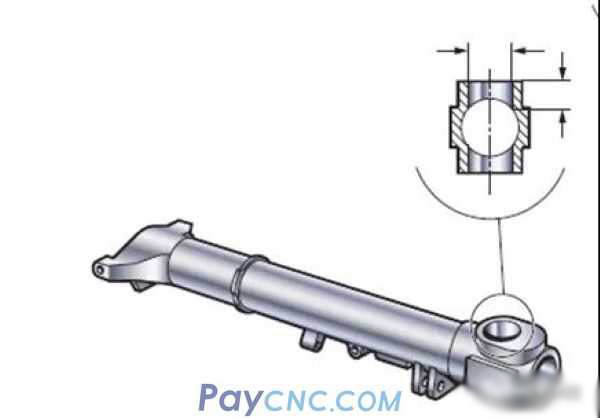For example, some time ago, a master made hole chamfering on a CNC milling machine. Although it was hole chamfering, he was doing aerospace products, which required relatively high quality. He used a chamfering tool and pierced it directly.The result of doing this:   1. Burrs are produced in the orifice, which affects product quality   2. The tool cost is a bit high because it uses a special chamfering tool   So, what are the ways to improve product quality?   From a programming point of view, one of the methods is: helical interpolation milling   1. The hole and chamfer can use a standard tool, both of which are milled by spiral interpolation milling, to avoid the generation of tool marks and burrs on the chamfer and hole.   2. It is required that the program has good versatility, and a program must satisfy different sizes of chamfers. Later, you can process holes of different sizes, tapered surfaces of different angles, and changes in tool diameters using this program.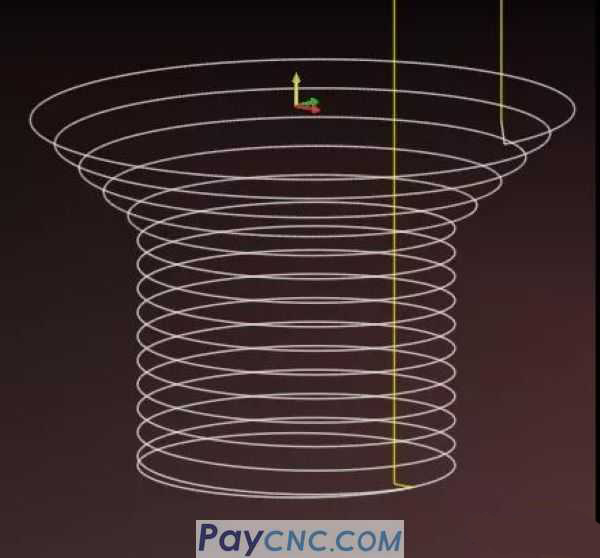How to realize the above two points through NC macro program?   This is the focus of my sharing today: logical reasoning   Take the spiral interpolation milling taper hole as an example (the spiral interpolation milling hole and the orifice rounding arc have been shared before and will not be repeated, if you need a private message, I will send you PPT)    The key point of logical reasoning is: deriving the mathematical relationship between variables   Let the mathematical relations participate in the calculations to complete the editing of the program!   To say this is vague, look at the diagram below and think about a question at the same time: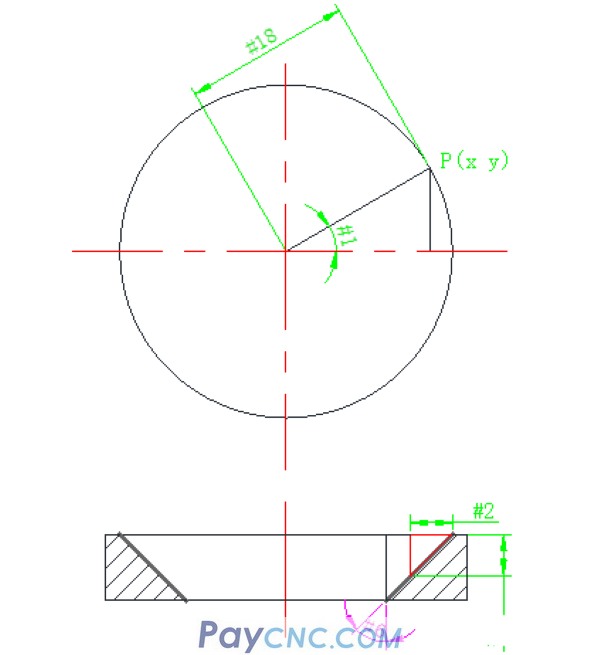If the set point P is any point on the arc, its X, Y, Z coordinates are represented by the macro variables recognized by the machine tool: #24, #25, #3 respectively.   How do you calculate the mathematical relationship of #24, #25, #3? What is the point coordinate of any point on the cone?    In a right-angled triangle, (as shown in the above diagram), according to the relationship between the angle #1 and the side of the trigonometric function, the following relationship can be derived:   #24=#18*COS[#1] #25= #18*SIN[#1]   Because of the rotation from angle #1, an arc with radius #18 will be drawn   This is a simple logical relationship. If the range of angle #1 is different, there will be corresponding arcs. such as: Let #1 increment from 0 to 180, and execute the following program to form a semicircle.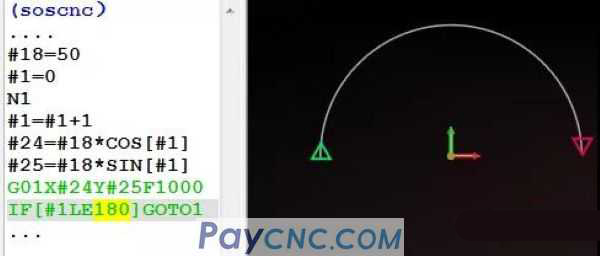such as: The value of #1 ranges from 0 to 270, and a 3/4 circle is formed by executing the following program.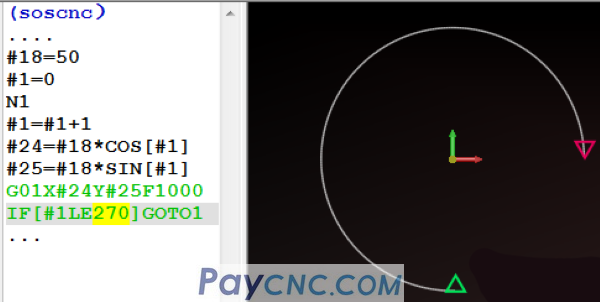such as: #1 From 0 to 360, it is a full circle.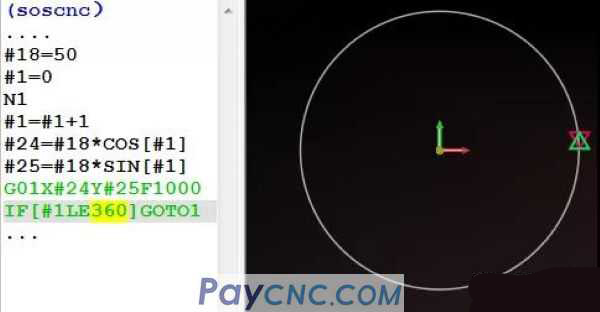Well, we want X, Y, Z three-axis linkage spiral interpolation milling cone, instead of walking on a plane arc     Imagine 2 questions: The first one: About the calculation of the relational expression of variable #3 (Z direction) (changes with the change of #1)   #1 self-increment, the range of 0～360 will be a whole circle, so the process of #1 self-increment will also gradually change the value in the Z direction. If I assign the value of #1 directly to #3, that is: #3=#1   Add a Z-#3 to G01X#24Y#25 in the above program, then a round spiral is completed!   So, let's see the value of #1 is directly assigned to #3, what will happen (in the Z direction)?   When #1=0, #3 is equal to 0 When #1=1, #3 is equal to 1. When #1 increases to 360, the value of #3 is also equal to 360   G01X#24Y#25Z-#3 That is, while walking a full circle, #3 (Z direction) drops -360   I want to drop 1mm in the Z direction every time I walk, what should I do?   The following relationship: #3=#1/360 It is easy to figure out, that is, to add a coefficient of 360 to #1   #3=#1/360 When #1 increases to 360, the value of #1/360 operation is also assigned to #3   For example, we look at the following program segment: #1 increases automatically, the range is 0～360, which is a circle, Z drops by 1mm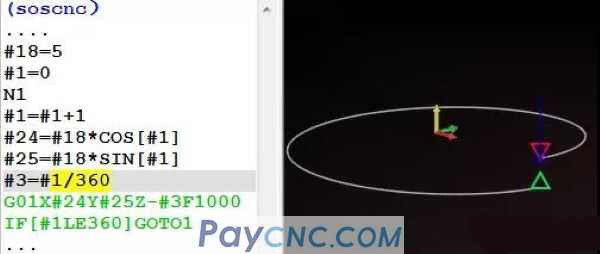#1 increases automatically, the range is from 0 to 720, which is 2 circles, Z has dropped by 2mm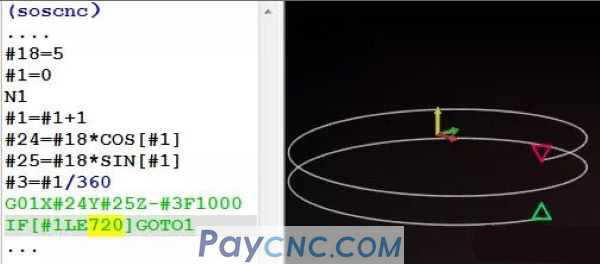2. On the calculation of any P point X Y on the cone   #24=#18*COS[#1] #25= #18*SIN[#1]   This is a milling garden, But the three-axis linkage spiral interpolation milling cone, as Z decreases, the radius (#18) will also change   How to change, see the picture below: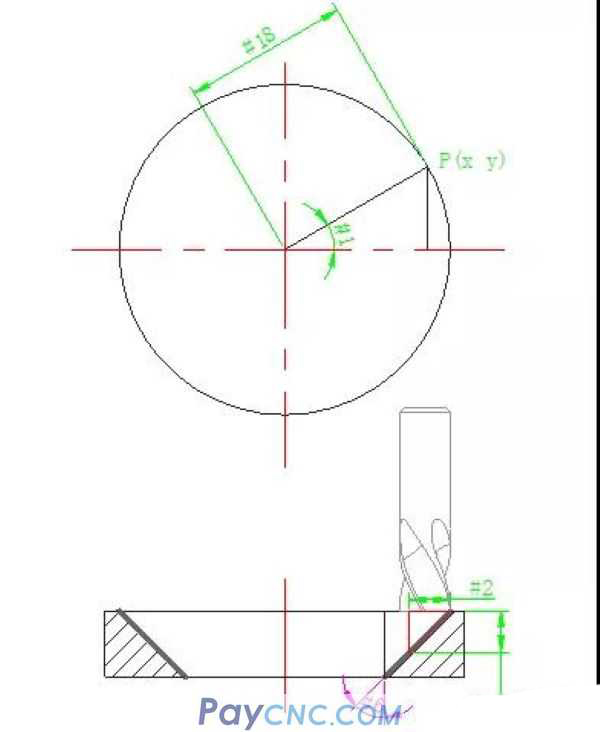TAN[#6]=#2/#3 can be calculated   #2= TAN[#6]* #3     OK, this #2 is the amount of change, which changes due to the change of #3 (the cutting depth in the Z direction)   The relational expression is: #2= TAN[#6]* #3 (Note: #6 represents taper variable)   Then the arithmetic formula of any P point X Y on the cone is:   #24=[#18-#2]*COS[#1] #25=[#18-#2]*SIN[#1]   This is the X and Y coordinates of any point P on the cone.     Calculate the X, Y and Z coordinates of any point P on the cone: #3=#1/360 (Each turn, the depth of Z's descent)   Then, the programming of the part can be completed soon.   such as #1 The range of self-increasing is 0～3600, which is 10 circles, Z has dropped by 10mm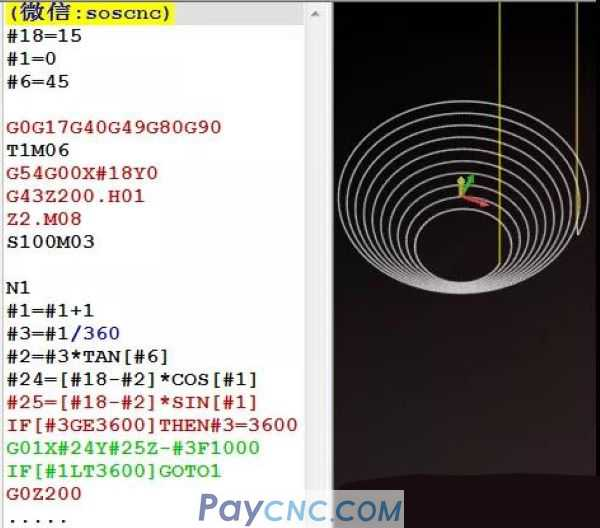Remarks: Variable #18 represents the radius value of the arc, and does not include the tool   When assigning a value to #18, it needs to be calculated: #18=(chamfer diameter-tool diameter)/2   I can set two variables separately, which represent the chamfer diameter and the tool diameter, as shown in the figure below#7 represents the diameter of the large end of the chamfer #20 represents the tool diameter   The above program becomes the following program: (assign #7, #20 instead of #18)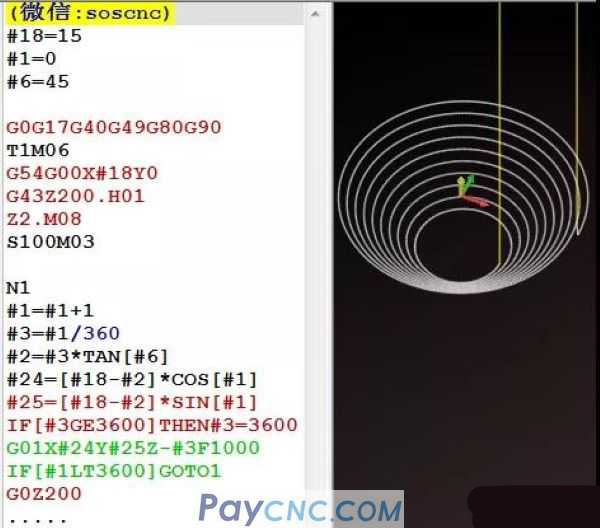According to the size of the chamfer, the diameter of the tool, and the size of the taper to be processed, assign values ​​to #7, #20, and #6 respectively.   OK, have you noticed that in the above program   1. The set variable #3=#1/360 The descending depth of each circle is fixed, namely 1mm   2. The total depth of the part in the Z direction is determined by #1   1 circle arc, the range of #1 is 0-360 2 arcs, the range of #1 is 0-720 ... 10 arcs, the range of #1 is 0-3600   From the above two points, the cutting depth of each circle and the total number of circles determine the total depth of the chamfer to be processed   In fact, we want to directly assign values ​​to variables to determine the total depth we want to process, not the number of turns. For example, set a variable #26 in the program to represent the total depth of the part in the Z direction.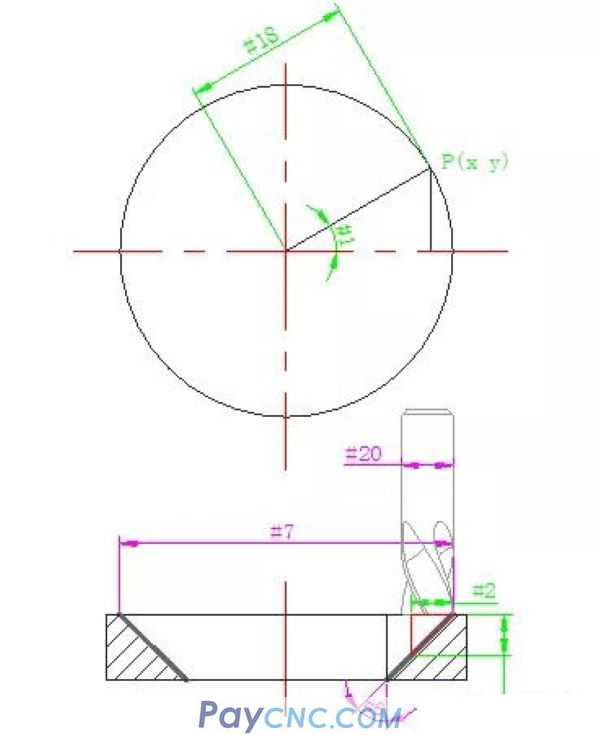Set a variable #4 to represent the cutting depth of each circle   Then #26/#4 represents the number of laps to go   #5=FUP[#26/#4] Round the number of turns   Remarks: FUP is a rounding function in a macro program. What does it mean?   If the result of the expression calculation has a decimal, the decimal part is changed to an integer 1, and added to the integer part   such as, #26=16 (part depth) #4=2.2 (cut depth per circle)   16/2.2=7.272   Then #5=FUP[#26/#4] The result of #5 is 8 (the value of #26/#4 operation, the decimal part becomes the integer 1, and it is added to the integer part)   Well, the reasoning between variables is shared here, I will give a program directly: